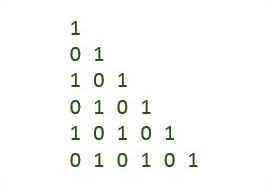# C Program to Print Binary Numbers Right Triangle Pyramid Pattern

Here is a C program to print right triangle of binary number. For a binary number right triangle of 6 rows, program's output should be:#### Required Knowledge

Algorithm to print triangle pattern of binary numbers
This program is similar to right triangle star pattern. The only difference is instead of printing star characters we will print binary digits.
• Take the number of rows(N) of right triangle as input from user using scanf function.
• Number of integers in Kth row is always K+1.
• We will use two for loops to print right triangle of binary numbers.
• Outer for loop will iterate N time(from 1 = 0 to N-1). Each iteration of outer loop will print one row of the pattern.
• Inside inner loop will toggle binary digits and print it. Each iteration of inner loop for Kth row will print K+1 alternating 0 and 1.

Here is the matrix representation of above mentioned pattern. The row numbers are represented by i whereas column numbers are represented by j.## C program to print right triangle pattern having same number in a row

```#include<stdio.h>

int main() {
int i, j, rows;
int count = 1;

printf("Enter the number of rows\n");
scanf("%d", &rows);

for (i = 0; i < rows; i++) {
for (j = 0; j <= i; j++) {
printf("%d ", count);
count = !count;
}
count = i % 2;
printf("\n");
}
return(0);
}
```
Output
```Enter the number of rows
5
1
0 1
1 0 1
0 1 0 1
1 0 1 0 1
```

Related Topics
 C program binary rectangle pattern C program natural number triangle pattern C program hollow diamond star pattern C program hollow pyramid star pattern C program diamond star pattern C program rectangle star pattern C program inverted right triangle pattern C program palindrome triangle pattern C program rhombus star pattern List of all C pattern programs